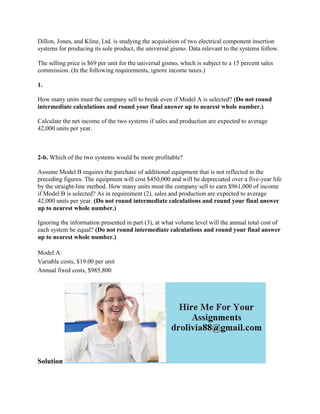Successfully reported this slideshow.

# Dillon- Jones- and Kline- Ltd- is studying the acquisition of two elec.docx×

# Dillon- Jones- and Kline- Ltd- is studying the acquisition of two elec.docx

Dillon, Jones, and Kline, Ltd. is studying the acquisition of two electrical component insertion systems for producing its sole product, the universal gismo. Data relevant to the systems follow.
The selling price is \$69 per unit for the universal gismo, which is subject to a 15 percent sales commission. (In the following requirements, ignore income taxes.)
1.
How many units must the company sell to break even if Model A is selected? (Do not round intermediate calculations and round your final answer up to nearest whole number.)
Calculate the net income of the two systems if sales and production are expected to average 42,000 units per year.

2-b. Which of the two systems would be more profitable?
Assume Model B requires the purchase of additional equipment that is not reflected in the preceding figures. The equipment will cost \$450,000 and will be depreciated over a five-year life by the straight-line method. How many units must the company sell to earn \$961,000 of income if Model B is selected? As in requirement (2), sales and production are expected to average 42,000 units per year. (Do not round intermediate calculations and round your final answer up to nearest whole number.)
Ignoring the information presented in part (3), at what volume level will the annual total cost of each system be equal? (Do not round intermediate calculations and round your final answer up to nearest whole number.)
Solution
Everything is correct in the above answer except for number 3
Only thing to add to get the right answer is the Target Profit.
((1,114,200+(450,000/5)) + 961,000) / (69*(1-.15)-11.80)
Which should give you: 46216 rounded to the nearest whole number
.

Dillon, Jones, and Kline, Ltd. is studying the acquisition of two electrical component insertion systems for producing its sole product, the universal gismo. Data relevant to the systems follow.
The selling price is \$69 per unit for the universal gismo, which is subject to a 15 percent sales commission. (In the following requirements, ignore income taxes.)
1.
How many units must the company sell to break even if Model A is selected? (Do not round intermediate calculations and round your final answer up to nearest whole number.)
Calculate the net income of the two systems if sales and production are expected to average 42,000 units per year.

2-b. Which of the two systems would be more profitable?
Assume Model B requires the purchase of additional equipment that is not reflected in the preceding figures. The equipment will cost \$450,000 and will be depreciated over a five-year life by the straight-line method. How many units must the company sell to earn \$961,000 of income if Model B is selected? As in requirement (2), sales and production are expected to average 42,000 units per year. (Do not round intermediate calculations and round your final answer up to nearest whole number.)
Ignoring the information presented in part (3), at what volume level will the annual total cost of each system be equal? (Do not round intermediate calculations and round your final answer up to nearest whole number.)
Solution
Everything is correct in the above answer except for number 3
Only thing to add to get the right answer is the Target Profit.
((1,114,200+(450,000/5)) + 961,000) / (69*(1-.15)-11.80)
Which should give you: 46216 rounded to the nearest whole number
.